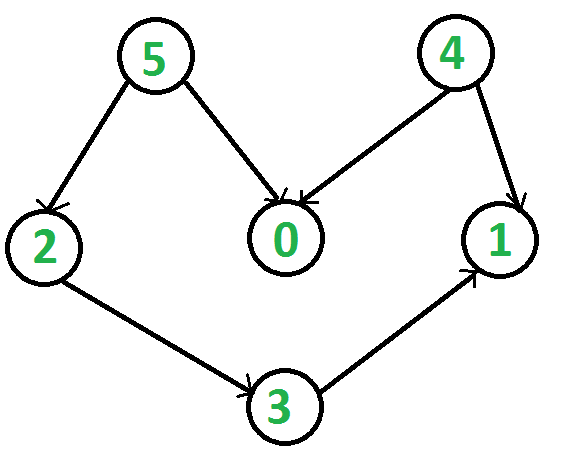2013-11-20

## O(V+E) 的算法topologicalsort

``````
#include <iostream>
#include <stdio.h>
#include <list>
#include <stack>

using namespace std;

class Graph {
int V;
void _topological_sort(int v, bool visited[], stack<int>& stack);
public:
Graph(int v);
~Graph();
void Topological_sort();
};

Graph::Graph(int v):V(v) {
}

Graph::~Graph() {
}

void Graph::addEdge(int v, int w) {
}

void Graph::_topological_sort(int v, bool visited[], stack<int>& stack) {
visited[v] = true;
int u = *it;
if(visited[u] == false)
_topological_sort(u, visited, stack);
}
stack.push(v);
}

void Graph::Topological_sort() {
bool visited[V];
stack<int> stack;
for(int i=0; i<V; i++)
visited[i] = false;
for(int i=V-1; i>=0; i--) {
if(visited[i] == false) {
_topological_sort(i, visited, stack);
}
}
while(!stack.empty()) {
int v = stack.top();
stack.pop();
std::cout << " " << v << " ";
}
std::cout << std::endl;
}

int main() {
Graph g(6);
cout << "Following is topological sort result: \n";
g.Topological_sort();
return 0;
}

``````

## 其他图算法的预处理

• DAG 的强连通分支问题
先得到拓扑排序，形成逆向图 (所有边与原来方向相反)，然后根据拓扑排序依次再进行 DFS。

• DAG 的最短路径问题，这可以在 O(V+E) 复杂度解决最短路径问题。同样类似的算法适用与 DAG 的最长路径问题，给定一个点求 DAG 中的各个点与给定点之间的最长路径。最长路径问题要比最短路径问题难，因为最长路径问题没有最优子结构，对于通用的图的最长路径算法还是 NP 难的问题